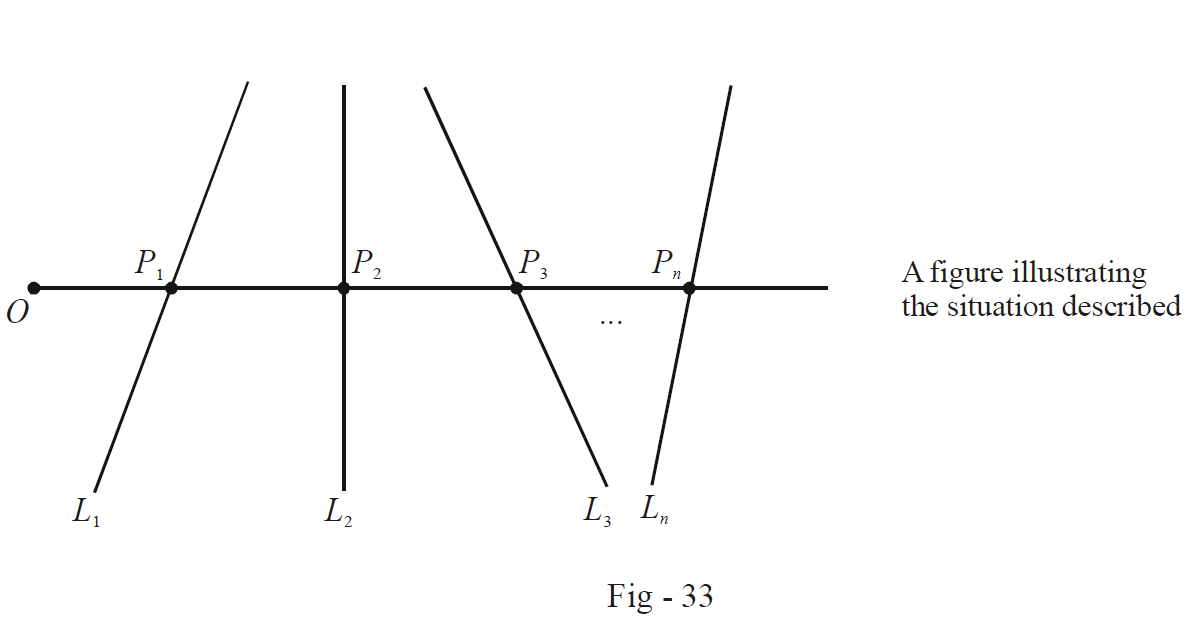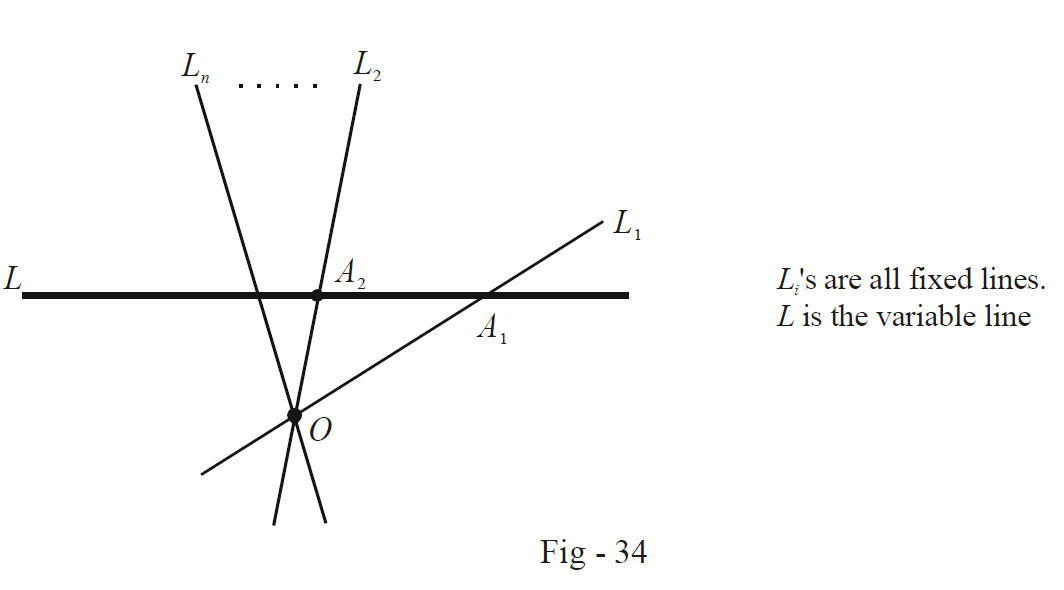# Straight Lines Set 1

Go back to  'Straight Lines'

Example – 1

Consider a fixed point O and n fixed straight lines. Through O, a (variable) line is drawn intersecting the fixed lines in  $${P_1},\,{P_2}.....,{P_n}.$$ On this variable line, a point P is taken such that

$\frac{n}{{OP}} = \frac{1}{{O{P_1}}} + \frac{1}{{O{P_2}}} + ..... + \frac{1}{{O{P_n}}}.$

Find the locus of P.

Solution:Assume the equations of the fixed lines to be

${L_i} \equiv {a_i}x + {b_i}y + {c_i} = 0, \;i = 1,\,2,\,.....n$

and the coordinates of the fixed point O to be $$(h,\,k).$$

Let the slope of the variable line be represented by $$\tan \theta .$$ Thus, the points $${P_i}$$ have the coordinates

${P_i} \equiv (h + O{P_i}\cos \theta ,k + O{P_i}\sin \theta ) \; i = 1,\,2.....n$

Since each $${P_i}$$ satisfies $${L_i},$$ we have

\begin{align}&{a_i}(h + O{P_i}\cos \theta ) + {b_i}(k + O{P_i}\sin \theta ) + {c_i} = 0 \\\Rightarrow \qquad &O{P_i} = \frac{{ - (h{a_i} + k{b_i} + {c_i})}}{{{a_i}\cos \theta + {b_i}\sin \theta }} \\ \end{align}

Assume the coordinates of P (whose locus we wish to determine) to be $$(x,\,y).$$ Thus, we have

$x = h + OP\cos \theta , \; y = k + OP\sin \theta \,\,\,\,\,\,\,\,\,\,\,\,\,\,\,\,\,\,\,\,\,\,\,\, \ldots \left( 1 \right)$

From the relation given in the equation, we have

\begin{align}&\frac{n}{{NP}} = \sum {\frac{1}{{O{P_i}}}} \\ \Rightarrow \qquad& \frac{n}{{NP}} = - \sum {\frac{{{a_i}\cos \theta + {b_i}\sin \theta }}{{h{a_i} + k{b_i} + {c_i}}}} \\ &\;\qquad= \left( { - \sum {\frac{{{a_i}}}{{h{a_i} + k{b_i} + {c_i}}}} } \right)\cos \theta + \left( { - \sum {\frac{{{b_i}}}{{h{a_i} + k{b_i} + {c_i}}}} } \right)\sin \theta \\ &\;\qquad = \lambda \cos \theta + \mu \sin \theta \,\,\,\,\,\,\,\,\,\,\,\,\,\,\,\,\,\,\left( \begin{gathered} {\text{These substitutions have}} \\ {\text{been doen for convenience}} \\ \end{gathered} \right) \\ \end{align}

From (1), we have

\begin{align}&\frac{n}{{OP}} = \frac{{\lambda (x - h)}}{{OP}} + \frac{{\mu (y - k)}}{{OP}} \\ \Rightarrow \qquad &\lambda x + \mu y - (\lambda h + \mu k + n) = 0\,\,\, \\\end{align}

This is the locus of the point P; it is evidently a straight line.

Example – 2

Lines are drawn to intersect n concurrent lines at the points  $${A_1},\,{A_2}.....,{A_n}$$ such that

$\sum\limits_{i = 1}^n {\frac{1}{{O{A_i}}} = {\text{constant}}}$

where O is the point of concurrency. Show that the variable lines all pass through a fixed point.

Solution: There’s no loss of generality in assuming O to be the origin since we are dealing only with lengths which are invariant with respect to the choice of the coordinate axes.The inclinations of the fixed lines can be assumed to be $${\theta _i}$$ so that the points $${A_i}$$ have the coordinates

${A_i} \equiv (O{A_i}\cos {\theta _i},\,O{A_i}\sin {\theta _i})$

Let the variable line have the equation

$ax + by + c = 0$

Since all the $${A_i}'s$$ lie on this line, we have

\begin{align}&aO{A_i}\cos {\theta _i} + bO{A_i}\sin {\theta _i} + c = 0 \\ \Rightarrow \qquad& O{A_i} = \frac{{ - c}}{{a\cos {\theta _i} + b\sin {\theta i}}}\,\,\,\,\,\,\,\,\,\,\,\,\,\,\,\,\,\,\,\,\,\,\,\,\,\,\,\,\,\,\,\,\,\,\,\,\,\,\,\,\,\,\,\,\,\,\,\, \ldots \left( 1 \right) \\ \end{align}

According to the condition specified in the question,

$\sum\limits_{i = 1}^n {\frac{1}{{O{A_i}}} = {\text{costant = }}K{\text{ (say)}}} \,\,\,\,\,\,\,\,\,\,\,\,\,\,\,\,\,\,\,\,\,\,\,\, \ldots \left( 2 \right)$

Thus, using (1) in (2), we have

\begin{align}&\sum\limits_{i = 1}^n {\frac{{a\cos {\theta _i} + b\sin {\theta _i}}}{{ - c}}{\text{ = }}K} \\ &a\left( {\frac{{\sum\limits_{i = 1}^n {\cos {\theta _i}} }}{K}} \right) + b\left( {\frac{{\sum\limits_{i = 1}^n {\sin {\theta _i}} }}{K}} \right) + c = 0\,\,\,\,\,\,\,\,\,\,\,\,\,\,\,\,\,\,\,\,\,\,\, \ldots \left( 3 \right) \\\end{align}

(3) shows that the variable line L always passes through the fixed point \begin{align}\left( {\frac{{\sum\limits_{i = 1}^n {\cos {\theta _i}} }}{K},\,\,\,\frac{{\sum\limits_{i = 10}^n {\sin {\theta _i}} }}{K}} \right).\end{align}

Download SOLVED Practice Questions of Straight Lines Set 1 for FREE
Straight Lines
grade 11 | Answers Set 2
Straight Lines
grade 11 | Questions Set 1
Straight Lines
grade 11 | Answers Set 1
Straight Lines
grade 11 | Questions Set 2